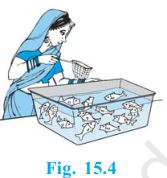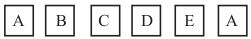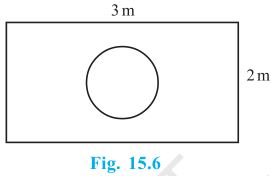# Class 10 Maths Chapter 15 Probability

NCERT Solutions for Class 10 Maths Chapter 15 Probability. Question Answer.

Page No: 308

Exercise 15.1

1. Complete the following statements:
(i) Probability of an event E + Probability of the event ‘not E’ = ___________ .
(ii) The probability of an event that cannot happen is __________. Such an event is called ________ .
(iii) The probability of an event that is certain to happen is _________ . Such an event is called _________ .
(iv) The sum of the probabilities of all the elementary events of an experiment is __________ .
(v) The probability of an event is greater than or equal to  and less than or equal to __________ .

(i) Probability of an event E + Probability of the event ‘not E’ = 1.
(ii) The probability of an event that cannot happen is 0. Such an event is called  impossible event.
(iii) The probability of an event that is certain to happen is 1. Such an event is called sure or certain event.
(iv) The sum of the probabilities of all the elementary events of an experiment is 1.
(v) The probability of an event is greater than or equal to 0 and less than or equal to 1.

2. Which of the following experiments have equally likely outcomes? Explain.
(i) A driver attempts to start a car. The car starts or does not start.
(ii) A player attempts to shoot a basketball. She/he shoots or misses the shot.
(iv) A baby is born. It is a boy or a girl.

(i) It does not have equally likely outcomes as it depends on various reasons like mechanical problems, fuels etc.
(ii) It does not have equally likely outcomes as it depends on the player how he/she shoots.
(iii) It has equally likely outcomes.
(iv)It has equally likely outcomes.

3. Why is tossing a coin considered to be a fair way of deciding which team should get the ball at the beginning of a football game?

Yes, tossing of a coin is a fair way of deciding which team should get the ball at the beginning of a football game because it has only two outcomes either head or tail. A coin is always unbiased.

4. Which of the following cannot be the probability of an event?
(A) 2/3     (B) -1.5     (C) 15%       (D) 0.7

The probability of an event is always greater than or equal to 0 and less than or equal to 1.
Thus, (B) -1.5 cannot be the probability of an event.

5. If P(E) = 0.05, what is the probability of ‘not E’?

P(E) = 0.05
also, P(E) + P(not E) = 1
⇒ P(not E) = 1 - P(E)
⇒ P(not E) = 1 - 0.05
⇒ P(not E) = 0.95

6. A bag contains lemon flavoured candies only. Malini takes out one candy without looking into the bag. What is the probability that she takes out
(i) an orange flavoured candy?
(ii) a lemon flavoured candy?

(i) Since the bag contains only lemon flavoured.
Therefor, No. of orange flavoured candies = 0
Probability of taking out orange flavoured candies = 0/1 = 0

(ii) The bag only have lemon flavoured candies.
Probability of taking out lemon flavoured candies = 1/1 = 1

7. It is given that in a group of 3 students, the probability of 2 students not having the same birthday is 0.992. What is the probability that the 2 students have the same birthday?

Let E be the event of having the same birthday.
P(E) = 0.992
⇒ P(E) + P(not E) = 1
⇒ P(not E) = 1 – P(E)
⇒ 1 - 0.992 = 0.008
The probability that the 2 students have the same birthday is 0.008

8. A bag contains 3 red balls and 5 black balls. A ball is drawn at random from the bag. What is the probability that the ball drawn is (i) red? (ii) not red?

No. of red balls = 3
No. of black balls = 5
Total no. of balls = 5+3 = 8
(i) Probability of drawing red balls = No. of red balls/Total no. of balls = 3/8

(ii) Probability of drawing black balls = No. of black balls/Total no. of balls = 5/8

9. A box contains 5 red marbles, 8 white marbles and 4 green marbles. One marble is taken out of the box at random. What is the probability that the marble taken out will be (i) red ? (ii) white ? (iii) not green?

No. of red marbles = 5
No. of white marbles =8
No. of green marbles = 4
Total no. of balls = 5+8+4 = 17

(i) Favourable no. of elementary events = 5
Probability of taking out red marble = 5/17

(ii) Favourable no. of elementary events = 8
Probability of taking out red marble = 8/17

(iii) Favourable no. of elementary events = 4
Probability of taking out red marble = 4/17

Page No: 309

10. A piggy bank contains hundred 50p coins, fifty ₹1 coins, twenty ₹2 coins and ten ₹5 coins. If it is equally likely that one of the coins will fall out when the bank is turned upside down, what is the probability that the coin (i) will be a 50 p coin ? (ii) will not be a ₹5 coin?

No. of 50p coins = 100
No. of ₹1 coins = 50
No. of ₹2 coins = 20
No. of ₹5 coins = 10
Total no. of coins = 100 + 50 + 20 + 10 = 180

(i) Favourable no. of elementary events = 100
Probability that it will be 50p coins = 100/180 = 5/9

(ii) Favourable no. of elementary events = 100+50+20 = 170
Probability that it will be 50p coins = 170/180 = 17/18

11. Gopi buys a fish from a shop for his aquarium. The shopkeeper takes out one fish at random from a tank containing 5 male fish and 8 female fish (see Fig. 15.4). What is the probability that the fish taken out is a male fish?No. of male fish in the tank = 5
no. of female fish in the tank = 8
Total number of fish in the tank = 5 + 8 = 13
Favourable number events = 5
Probability of taking out a male fish = 5/13

12. A game of chance consists of spinning an arrow which comes to rest pointing at one of the numbers 1, 2, 3, 4, 5, 6, 7, 8 (see Fig. 15.5), and these are equally likely outcomes. What is the probability that it will point at
(i) 8 ?
(ii) an odd number?
(iii) a number greater than 2?
(iv) a number less than 9?Possible no. of events = 8
(i) Favourable number of events = 1
Probability that it will point at 8 = 1/8

(ii) Odd numbers = 1, 3, 5 and 7
Favourable number of events = 4
Probability that it will be an odd number = 4/8 = 1/2

(iii) Numbers greater than 2 = 3, 4, 5, 6, 7 and 8
Favourable number of events = 6
Probability that a number greater than 4 = 6/8 = 3/4

(iv) Numbers less than 9 = 1,2,3,4,5,6,7,8
Favourable number of events = 8
Probability that a number less than 9 = 8/8 = 1

13. A die is thrown once. Find the probability of getting
(i) a prime number; (ii) a number lying between 2 and 6; (iii) an odd number.

Possible numbers of events on throwing a dice = 6
Numbers on dice = 1,2,3,4,5 and 6

(i) Prime numbers = 2, 3 and 5
Favourable number of events = 3
Probability that it will be a prime number = 3/6 = 1/2

(ii) Numbers lying between 2 and 6 = 3, 4 and 5
Favourable number of events = 3
Probability that a number between 2 and 6 = 3/6 = 1/2

(iii) Odd numbers = 1, 3 and 5
Favourable number of events = 3
Probability that it will be an odd number = 3/6 = 1/2

14. One card is drawn from a well-shuffled deck of 52 cards. Find the probability of getting
(i) a king of red colour (ii) a face card (iii) a red face card (iv) the jack of hearts (v) a spade (vi) the queen of diamonds

Possible numbers of events = 52

(i) Numbers of king of red colour = 2
Probability of getting a king of red colour = 2/52 = 1/26

(ii) Numbers of face cards = 12
Probability of getting a face card = 12/52 = 3/13

(iii) Numbers of red face cards = 6
Probability of getting a king of red colour = 6/52 = 3/26

(iv) Numbers of jack of hearts =1
Probability of getting a king of red colour = 1/52

(v) Numbers of king of spade = 13
Probability of getting a king of red colour = 13/52 = 1/4

(vi) Numbers of queen of diamonds = 1
Probability of getting a king of red colour = 1/52

15. Five cards the ten, jack, queen, king and ace of diamonds, are well-shuffled with their face downwards. One card is then picked up at random.
(i) What is the probability that the card is the queen?
(ii) If the queen is drawn and put aside, what is the probability that the second card picked up is (a) an ace? (b) a queen?

Total numbers of cards = 5

(i) Numbers of queen = 1
Probability of picking a queen = 1/5

(ii) When queen is drawn and put aside then total numbers of cards left is 4
(a) Numbers of ace = 1
Probability of picking an ace = 1/4
(a) Numbers of queen = 0
Probability of picking a queen = 0/4 = 0

16. 12 defective pens are accidentally mixed with 132 good ones. It is not possible to just look at a pen and tell whether or not it is defective. One pen is taken out at random from this lot. Determine the probability that the pen taken out is a good one.

Numbers of defective pens = 12
Numbers of good pens = 132
Total numbers of pen = 132 + 12 = 144 pens
Favourable number of events = 132
Probability of getting a good pen = 132/144 = 11/12

17. (i) A lot of 20 bulbs contain 4 defective ones. One bulb is drawn at random from the lot. What is the probability that this bulb is defective?
(ii) Suppose the bulb drawn in (i) is not defective and is not replaced. Now one bulb is drawn at random from the rest. What is the probability that this bulb is not defective?

(i) Total numbers of bulbs = 20
Numbers of defective bulbs = 4
Probability of getting a defective bulb = 4/20 = 1/5

(ii) One non defective bulb is drawn in (i) then the total numbers of bulb left is 19
Total numbers of events = 19
Favourable numbers of events =  19 - 4 = 15
Probability that the bulb is not defective = 15/19

18. A box contains 90 discs which are numbered from 1 to 90. If one disc is drawn at random from the box, find the probability that it bears (i) a two-digit number (ii) a perfect square number (iii) a number divisible by 5.

Total numbers of discs = 50

(i) Total numbers of favourable events = 81
Probability that it bears a two-digit number = 81/90 = 9/10

(ii) Perfect square numbers = 1, 4, 9, 16, 25, 36, 49, 64 and 81
Favourable numbers of events = 9
Probability of getting a perfect square number = 9/90 = 1/10

(iii) Numbers which are divisible by 5 = 5, 10, 15, 20, 25, 30, 35, 40, 45, 50, 55, 60, 65, 70, 75, 80, 85 and 90
Favourable numbers of events = 18
Probability of getting a number divisible by 5 = 18/90 = 1/5

Page No: 310

19. A child has a die whose six faces show the letters as given below:The die is thrown once. What is the probability of getting (i) A? (ii) D?

Total numbers of events = 6

(i) Total numbers of faces having A on it = 2
Probability of getting A = 2/6 = 1/3

(ii) Total numbers of faces having D on it = 1
Probability of getting A = 1/6

20. Suppose you drop a die at random on the rectangular region shown in Fig. 15.6. What is the probability that it will land inside the circle with diameter 1m?Area of the rectangle = (3 × 2) m2 = 6m2
Area of the circle = πr2 = π(1/2)2 m2  = π/4 m2
Probability that die will land inside the circle = (π/4) × 1/6 = π/24

21. A lot consists of 144 ball pens of which 20 are defective and the others are good. Nuri will buy a pen if it is good, but will not buy if it is defective. The shopkeeper draws one pen at random and gives it to her. What is the probability that
(ii) She will not buy it?

Total numbers of pens = 144
Numbers of defective pens = 20
Numbers of non defective pens = 144 - 20 = 124

(i) Numbers of favourable events = 124
Probability that she will buy it = 124/144 = 31/36

(ii) Numbers of favourable events = 20
Probability that she will not buy it = 20/144 = 5/36

22. Refer to Example 13. (i) Complete the following table:(ii) A student argues that ‘there are 11 possible outcomes 2, 3, 4, 5, 6, 7, 8, 9, 10, 11 and 12. Therefore, each of them has a probability 1/11. Do you agree with this argument? Justify your answer.

Events that can happen on throwing two dices are:
(1, 1), (1, 2), (1, 3), (1, 4), (1, 5), (1, 6)
(2, 1), (2, 2), (2, 3), (2, 4), (2, 5), (2, 6)
(3, 1), (3, 2), (3, 3), (3, 4), (3, 5), (3, 6)
(4, 1), (4, 2), (4, 3), (4, 4), (4, 5), (4, 6)
(5, 1), (5, 2), (5, 3), (5, 4), (5, 5), (5, 6)
(6, 1), (6, 2), (6, 3), (6, 4), (6, 5), (6, 6)
Total numbers of events : 6 × 6 = 36

(i) To get sum as 2, possible outcomes = (1,1)
To get sum as 3, possible outcomes = (1,2) and (2,1)
To get sum as 4, possible outcomes = (1,3); (3,1);  and (2,2)
To get sum as 5, possible outcomes = (1,4); (4,1); (2,3);  and (3,2)
To get sum as 6, possible outcomes = (1,5); (5,1); (2,4); (4,2);  and (3,3)
To get sum as 7, possible outcomes = (1,6); (6,1); (5,2); (2,5); (4,3);  and (3,4)
To get sum as 8, possible outcomes = (2,6); (6,2); (3,5); (5,3);  and (4,4)
To get sum as 9, possible outcomes = (3,6); (6,3); (4,5);  and (5,4)
To get sum as 10, possible outcomes = (4,6); (6,4) and (5,5)
To get sum as 11, possible outcomes = (5,6) and (6,5)
To get sum as 12, possible outcomes = (6,6)

 Event:Sum on 2 dice 2 3 4 5 6 7 8 9 10 11 12 Probability 1/36 2/36 3/36 4/36 5/36 6/36 5/36 4/36 3/36 2/36 1/36

(ii) No, i don't agree with the argument. It is already justified in (i).

23. A game consists of tossing a one rupee coin 3 times and noting its outcome each time. Hanif wins if all the tosses give the same result i.e., three heads or three tails, and loses otherwise. Calculate the probability that Hanif will lose the game.

Events that can happen in tossing 3 coins = HHH, HHT, HTH, THH, TTH, HTT, THT, TTT
Total number of events = 8
Hinif will lose the game if he gets HHT, HTH, THH, TTH, HTT, THT
Favourable number of elementary events = 6
Probability of losing the game = 6/8 = 3/4

24. A die is thrown twice. What is the probability that
(i) 5 will not come up either time? (ii) 5 will come up at least once?
[Hint : Throwing a die twice and throwing two dice simultaneously are treated as the same experiment]

(i) Consider the following events.
A = first throw shows 5,
B = second throw shows 5
P(A) = 6/36, P(B) = 6/36 and P(notB) = 5/6
⇒ P(notA) = 1– 6/36 = 30/36 = 5/6
Required probability = 5/6 × 5/6 = 25/36

(ii) Number of events when 5 comes at least once = 11
Probability = 11/36

Page No: 311

25. Which of the following arguments are correct and which are not correct? Give reasons for your answer.
(i) If two coins are tossed simultaneously there are three possible outcomes—two heads, two tails or one of each. Therefore, for each of these outcomes, the probability is 1/3
(ii) If a die is thrown, there are two possible outcomes—an odd number or an even number. Therefore, the probability of getting an odd number is 1/2